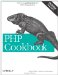# Recipe 21.2. Timing Program Execution

#### 21.2.1. Problem

You have a block of code and you want to profile it to see how long each statement takes to execute.

#### 21.2.2. Solution

Use the PEAR Benchmark module:

`<?php require_once 'Benchmark/Timer.php'; \$timer =& new Benchmark_Timer(true); \$timer->start(); // some setup code here \$timer->setMarker('setup'); // some more code executed here \$timer->setMarker('middle'); // even yet still more code here \$timer->setmarker('done'); // and a last bit of code here \$timer->stop(); \$timer->display(); ?>`

#### 21.2.3. Discussion

Calling setMarker( ) records the time. The display( ) method prints out a list of markers, the time they were set, and the elapsed time from the previous marker:

`------------------------------------------------------------- marker    time index            ex time               perct ------------------------------------------------------------- Start     1029433375.42507400   -                       0.00% ------------------------------------------------------------- setup     1029433375.42554800   0.00047397613525391    29.77% ------------------------------------------------------------- middle    1029433375.42568700   0.00013899803161621     8.73% ------------------------------------------------------------- done      1029433375.42582000   0.00013303756713867     8.36% ------------------------------------------------------------- Stop      1029433375.42666600   0.00084602832794189    53.14% ------------------------------------------------------------- total     -                     0.0015920400619507    100.00% -------------------------------------------------------------`

The Benchmark module also includes the Benchmark_Iterate class, which can be used to time many executions of a single function:

`<?php require 'Benchmark/Iterate.php'; \$timer =& new Benchmark_Iterate; // a sample function to time function use_preg(\$ar) {     for (\$i = 0, \$j = count(\$ar); \$i < \$j; \$i++) {         if (preg_match('/gouda/',\$ar[\$i])) {             // it's gouda         }     } } // another sample function to time function use_equals(\$ar) {     for (\$i = 0, \$j = count(\$ar); \$i < \$j; \$i++) {         if ('gouda' == \$ar[\$i]) {             // it's gouda         }     } } // run use_preg() 1000 times \$timer->run(1000,'use_preg',                 array('gouda','swiss','gruyere','muenster','whiz')); \$results = \$timer->get(); print "Mean execution time for use_preg(): \$results[mean]\n"; // run use_equals() 1000 times \$timer->run(1000,'use_equals',                 array('gouda','swiss','gruyere','muenster','whiz')); \$results = \$timer->get(); print "Mean execution time for use_equals(): \$results[mean]\n"; ?>`

The Benchmark_Iterate::get( ) method returns an associative array. The mean element of this array holds the mean execution time for each iteration of the function. The iterations element holds the number of iterations. The execution time of each iteration of the function is stored in an array element with an integer key. For example, the time of the first iteration is in \$results, and the time of the 37th iteration is in \$results.

To automatically record the elapsed execution time after every line of PHP code, use the declare construct and the ticks directive:

`function profile(\$display = false) {     static \$times;     switch (\$display) {     case false:         // add the current time to the list of recorded times         \$times[] = microtime();         break;     case true:         // return elapsed times in microseconds         \$start = array_shift(\$times);         \$start_mt = explode(' ', \$start);         \$start_total = doubleval(\$start_mt) + \$start_mt;         foreach (\$times as \$stop) {             \$stop_mt = explode(' ', \$stop);             \$stop_total = doubleval(\$stop_mt) + \$stop_mt;             \$elapsed[] = \$stop_total - \$start_total;         }         unset(\$times);         return \$elapsed;         break;     } } // register tick handler register_tick_function('profile'); // clock the start time profile(); // execute code, recording time for every statement execution declare (ticks = 1) {     foreach (\$_SERVER['argv'] as \$arg) {         print strlen(\$arg);     } } // print out elapsed times \$i = 0; foreach (profile(true) as \$time) {     \$i++;     print "Line \$i: \$time\n"; }`

The ticks directive allows you to execute a function on a repeatable basis for a block of code. The number assigned to ticks is how many statements go by before the functions that are registered using register_tick_function( ) are executed.

In the previous example, we register a single function and have the profile( ) function execute for every statement inside the declare block. If there are two elements in \$_SERVER['argv'], profile( ) is executed four times: once for each time through the foreach loop, and once each time the print strlen(\$arg) line is executed.

You can also set things up to call two functions every three statements:

`register_tick_function('profile'); register_tick_function('backup'); declare (ticks = 3) {     // code... }`

You can also pass additional parameters into the registered functions, which can be object methods instead of regular functions:

`// pass "parameter" into profile() register_tick_function('profile', 'parameter'); // call \$car->drive(); \$car = new Vehicle; register_tick_function(array(\$car, 'drive'));`

If you want to execute an object method, pass the object and the name of the method encapsulated within an array. This lets the register_tick_function( ) know you're referring to an object instead of a function.

Call unregister_tick_function( ) to remove a function from the list of tick functions:

`unregister_tick_function('profile');`

http://pear.php.net/package/Benchmark for information on the PEAR Benchmark class; documentation on register_tick_function( ) at http://www.php.net/register-tick-function, unregister_tick_function( ) at http://www.php.net/unregister-tick-function, and declare at http://www.php.net/declare.PHP Cookbook: Solutions and Examples for PHP Programmers
ISBN: 0596101015
EAN: 2147483647
Year: 2006
Pages: 445

Similar book on Amazon